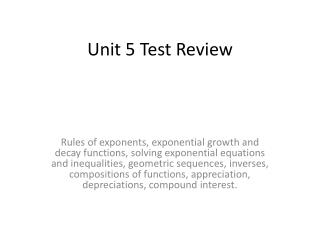DownloadDownload PresentationUnit 5 Test Review

# Unit 5 Test Review

Télécharger la présentation## Unit 5 Test Review

- - - - - - - - - - - - - - - - - - - - - - - - - - - E N D - - - - - - - - - - - - - - - - - - - - - - - - - - -
##### Presentation Transcript

1. Unit 5 Test Review Rules of exponents, exponential growth and decay functions, solving exponential equations and inequalities, geometric sequences, inverses, compositions of functions, appreciation, depreciations, compound interest.

2. Question 1 • Simplify

3. Question 2 • Simplify

4. Question 3 • What is the domain and range for the function: y = 4(2)x + 6?

5. Question 4 • Shrink by a SF of ½, shift to the left 7, shift down 3 Go to #14 • Stretch by a SF of ½, shift to the right 7, shift down 3 Go to #7 • Shrink by a SF of ½ , reflect across the x-axis, shift to the left 7, shift down 3 Go to #6 • Shrink by a SF of ½, reflect across the x-axis, shift to the left 3, shift up 7 Go to #9

6. Question 5 • What is the common ratio in the following geometric sequence:

7. Question 6 • If a3=12 and r= 3, what is a1?

8. Question 7 • Write the rule for the geometric sequence if a3 = 8 and a5 = 288.

9. Question 8 • If f(x) = x2 + 3 and g(x) = x -5 what is f(g(x))? • x2 – 2 Go to # 10 • x2 - 10x + 28 Go to # 2 • x2 + x - 2 Go to # 15 • x3 - 5x2 +3x - 15 Go to # 18

10. Question 9 • What is the inverse of the function

11. Question 10 • Solve for x: 3x-2 = 272x-1

12. Question 11 • A new car is purchased for \$40,000. If the value of the car depreciated at 10% each year, when will the car be worth \$20,000? • About 60.5 years Go to #14 • About 6.5 years Go to #9 • About 10 years Go to #6 • About 5 years Go to #2

13. Question 12 • A ring is purchased for \$1,000. The value of the ring appreciates 5% each year. How much will the ring be worth after 10 years? • \$162,051 Go to #3 • \$598.74 Go to #7 • \$57,665.04 Go to #15 • \$1,628.89 Go to #10

14. Question 13 • \$2,000 is deposited into a savings account that is compound quarterly at 3%. How much will be in the bank after 5 years? • \$2,318.55 Go to #4 • \$2,322.37 Go to #1 • \$8,495.70 Go to #10 • \$2,076.13 Go to #15

15. Question 14 • What is the rate of change for over the interval

16. Question 15 • Solve the inequality:

17. Question 16 • If and , what is f(x)-g(x)? • x – 1 Go to #11 • x2 - x - 1 Go to #4 • x2 – x + 5 Go to #3 • x2 – 1 Go to #17

18. Question 17 • Which of the following statements is true if f(x) = 3x2 + 4, g(x) = x–2, & h(x) = 3x2 - 12x+ 16 • h(x) = f(x) – g(x) Go to #9 • h(x) = f(g(x)) Go to #5 • h(x) = g(f(x)) Go to #11 • h(x) = f(x) + g(x) Go to #2

19. Question 18 • Which of the following points would be on the graph of

20. Answer key • 1. B 8. B 15. C • 2. C 9. C 16. C • 3. A 10. A 17. B • 4. C 11. B 18. C • 5. D 12. D • 6. A 13. B • 7. D 14. A